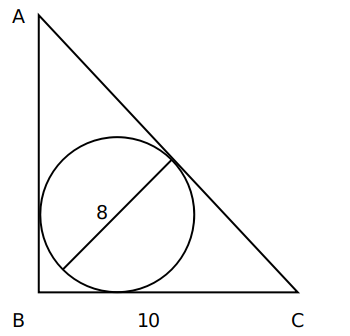Question 58

# A circle of diameter 8 inches is inscribed in a triangle ABC where $$\angle ABC = 90^\circ$$. If BC = 10 inches then the area of the triangle in square inches is

SolutionWe know that Inradius=$$\frac{\left(Perpendicular+Base-Hypotenuse\right)}{2}$$

$$4=\frac{\left(p+10-h\right)}{2}$$

h-p = 2 or h= p+2.

Now, $$p^2+100\ =\ h^2$$

$$p^2+100\ =\ \left(p+2\right)^2$$

$$p^2+100\ =\ p^2+4p+4$$

4p = 96

p=24.

Hence, Area = $$\frac{1}{2}\times\ 10\times\ 24\ =\ 120$$.